# Calculus with MATLAB

copyright © 2000 by Paul Green and Jonathan Rosenberg

In this M-book, we will try to present some of the central ideas involved in doing calculus with  MATLAB.  The central concept is that of a function. We will discuss first the representation of functions and then the ways of accomplishing the things we want to do with them. These fall into three broad categories: symbolic computation, numerical computation, and plotting, and we will deal with each of them in turn.  We will try to provide concrete illustrations of each of the concepts involved as we go along. For the present, we will confine outselves to functions of one variable. Later, we will need to discuss MATLAB's routines for dealing with functions of several variables.

# Functions and Symbolic Differentiation

There are two distinct but related notions of function that are important in Calculus. One is a symbolic expression such as sin(x) or x2. The other is a rule (algorithm) for producing a numerical output from a given numerical input or set of numerical inputs. This definition is more general; for example, it allows us to define a function f(x) to be x2 in case x is negative or 0, and sin(x) in case x is positive. On the other hand, any symbolic expression implies a rule for evaluation. That is, if we know that f(x) = x2, we know that f(4) = 42 = 16. In MATLAB, the fundamental difference between a function and a symbolic expression is that a function can be called with arguments and a symbolic expression cannot. On the other hand, a symbolic expression can be differentiated symbolically, while a function cannot. MATLAB functions can be created in two ways: as function m-files, and as inline functions. A typical way to define a symbolic expression is as follows:

syms x

f=x^2-sin(x)

f =

x^2-sin(x)

The next two lines will show that we can differentiate f, but we cannot evaluate it, at least in the obvious way. Notice that MATLAB recognizes the variable. In the case of several symbolic variables, we can specify the one with respect to which we want to differentiate.

ans =

2*x-cos(x)

We can evaluate f(4) by substituting 4 for x, or in other words, by typing

ans =

16.7568

We can also turn f into an inline function with the command:

fin =

Inline function:

fin(x) = x^2-sin(x)

What's going on here is that the inline command requires a string as an input, and char turns f from a symbolic expression to the string 'x^2-sin(x)'.  (If we had simply typed fin=inline(f) we'd get an error message, since f is not a string.) The inline function fin now accepts an argument:

ans =

16.7568

Similarly we can construct a function from the derivative of f :

fxin =

Inline function:

fxin(x) = 2*x-cos(x)

The matlab function 'char' replaces its argument by the string that represents it, thereby making it available to functions such as 'inline' that demand strings as input. However, when 'char' is applied to a symbolic expression, the result is still symbolic and can be symbolically differentiated.

ans =

2*x-cos(x)

The other way to create a function that can be evaluated is to write a function m-file. This is the primary way to define a function in most applications of MATLAB, although we shall be using it relatively seldom. The m-file is cannot be created inside a document such as this, but must be separate. We have written the mfile fun1.m; you can see the text by following the hyperlink. It is a good practice to create such links (using control-K) when creating m-files in conjunction with MATLAB M-books.

ans =

16.7568

# Problem 1:

Let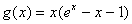.

a)      Enter the formula for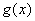as a symbolic expression.

b)      Obtain and name a symbolic expression for.

c)      Evaluate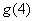and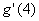, using "subs".

d)      Evaluateand, by creating inline functions.

e)      Evaluateby creating an m-file.

# Graphics and Plotting.

One of the things we might want to do with a function is plot its graph. MATLAB's most elementary operation is to plot a point with specified coordinates.

The output from this command is the faint blue dot in the center of the figure. The way MATLAB plots a curve is to plot a sequence of dots connected by line segments. The input for such a plot consists of two vectors (lists of numbers). The first argument is the vector of x-coordinates and the second is the vector of y-coordinates. MATLAB connects dots whose coordinates appear in consecutive positions in the input vectors. Let us plot the function we defined in the previous section. First we prepare a vector of x-coordinates

X1 =

Columns 1 through 7

-2.0000   -1.5000   -1.0000   -0.5000         0    0.5000    1.0000

Columns 8 through 9

1.5000    2.0000

X1 is now a vector of nine components, starting with -2 and proceeding by increments of .5 to 2. We must now prepare an input vector of y-coordinates by applying our function to the x-coordinates. Our function fin will accomplish this, provided that "vectorize" it, i.e., we redefine it to be able to operate on vectors.

The matlab function vectorize replaces *, ^, and / by  .*, .^, and ./ respectively. The significance of this is that MATLAB works primarily with vectors and matrices, and its default interpretation of multiplication, division, and exponentiation is as matrix operations. The dot before the operation indicates that it is to be performed entry by entry, even on matrices, which must therefore be the same shape. There is no difference between the dotted and undotted operations for numbers. However vectorized expressions are not interpereted as symbolic, in the sense that they cannot usually be symbolically differentiated. Fortunately, it is never necessary to do so.

fin =

Inline function:

fin(x) = x.^2-sin(x)

Y1 =

Columns 1 through 7

4.9093    3.2475    1.8415    0.7294         0   -0.2294    0.1585

Columns 8 through 9

1.2525    3.0907

This plot is somewhat crude; we can see the corners. To remedy this we will decrease the step size. We will also insert semicolons after the definitions of X1 and Y1 to suppress the output.

X1=-2:.02:2;

Y1=fin(X1);

plot(X1,Y1)

Actually, the plotting of a symbolic function of one variable can be accomplished much more easily with the command ezplot, as in the M-Book Introduction to MATLAB. However, plot allows more direct control over the plotting process, and enables one to modify the color, appearance of the curves, etc.  (Type help plot for more on this.)

# Problem 2:

Let the function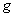be as in Problem 1.

a)      Plotfor x between –3 and 3 using plot and a small enough step size to make the resulting curve reasonably smooth.

b)      Plotusing ezplot.

c)      Combine the previous plot with a plot of.  Plot enough of both curves so that you can be certain that the plot shows all points of intersection.

# Solving Equations

The plot of f indicates that there are two solutions to the equation f(x)=0, one of which is clearly 0. We have both solve, a symbolic equation solver, and fzero, a numerical equations solver, at our disposal. Let us illustrate solve first, but with an easier example.

g =

x^2-7*x+2

groots =

[ 7/2+1/2*41^(1/2)]

[ 7/2-1/2*41^(1/2)]

Here solve finds all the roots it can, and reports them as the components of a column vector. Ordnarily, solve will solve for x, if present, or for the variable alphabetically closest to x otherwise. This can be overridden by specifying the variable to solve for. Notice also that the first argument to solve is a symbolic expression, which solve sets equal to 0.

syms y

solve(x^2+y^2-4,y)

ans =

[  (-x^2+4)^(1/2)]

[ -(-x^2+4)^(1/2)]

Now let us try solve on our function f.

froots =

0

Here solve found the root at 0, but not the other one. Let us try fzero, which solves equations numerically, starting at a given initial value of the variable. The command fzero will not accept f as an argument, but it will accept char(f) or (either version of)  fin, and find the second root. It will also accept the name of our mfile 'fun1' (with the quotes) or the construct @fun1 (the @ is a marker for a function name):

Zero found in the interval: [0.70949, 0.89051].

newfroot =

0.8767

Zero found in the interval: [0.70949, 0.89051].

newfroot =

0.8767

Zero found in the interval: [0.70949, 0.89051].

newfroot =

0.8767

# Problem 3:

Letbe as in Problems 1 and 2.

a)      Referring to the plot in part  c) of Problem 2, estimate those values of x for which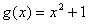.

b)      Use solve to obtain more precise values and check the equationfor those values.

# Symbolic and Numerical Integration

We have not yet dealt with integration. MATLAB has a symbolic integrator, called int, that will easily integrate f.

intsf =

5/3+cos(2)

However, if we replace f by the function h, defined by

h =

(x^2-sin(x^4))^(1/2)

int will be unable to evaluate the integral.

Warning: Explicit integral could not be found.

> In C:\MATLABR11\toolbox\symbolic\@sym\int.m at line 58

ans =

int((x^2-sin(x^4))^(1/2),x = 0 .. 2)

However, if we type double (standing for double-precision number) in front of the integral expression, MATLAB will return the result of a numerical integration.

Warning: Explicit integral could not be found.

> In C:\MATLABR11\toolbox\symbolic\@sym\int.m at line 58

ans =

1.7196

We can check the plausibility of this answer by plotting h between 0 and 2 and estimating the area under the curve.

The numerical value returned by MATLAB is somewhat less than half the area of a square 2 units on a side. This appears to be consistent with our plot.

The numerical integration invoked by the combination of double and int is native, not to MATLAB, but to MAPLE, from which MATLAB's symbolic routines are borrowed.  MATLAB also has its own numerical integrator called quadl. (The name comes from quadrature, an old word for numerical integration, and the "l" has something to do with the algorithm used, though there's no need for us to discuss it here). The routines double(int(…)) and quadl(…) give slighly different answers, though usually they agree to several decimal places.

ans =

1.7196

# Problem 4:

Letbe as in previous problems.

a)      Evaluate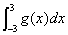symbolically, using int, and numerically using quadl. Compare your answers.

b)      Evaluate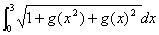, both using int and double, and using quadl.

c)      Check the plausibility of your answers to part b) by means of an appropriate plot.

# Additional Problems

1. Let f(x)=x5+3x4-3x+7

a)      Plot f between x = -1 and x = 1.

b)      Compute the derivative of f.

c)      Use solve to find all critical points of f.

d)      Find the extreme values of f on the interval [-1,1].  Hint: solve will store the critical points in a vector, which you cannot use unless you name it. You can compute the values of f at all critical points at once if you use an inline vectorized form of the derivative. Remember that you also need the values of f at 1 and –1.

2. Find all real roots of the equation x = 4 sin(x). You will need to use fzero.

3. Evaluate each of the following integrals, using both int and quadl (or quad8, if you're using MATLAB 5).

a)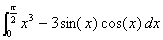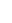b)